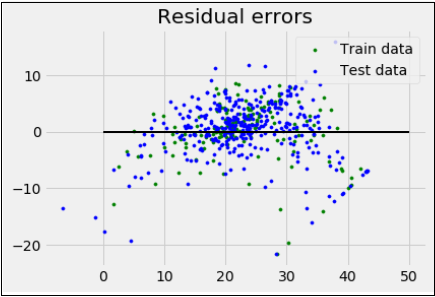# ML - Multiple Linear Regression

It is the extension of simple linear regression that predicts a response using two or more features. Mathematically we can explain it as follows −

Consider a dataset having n observations, p features i.e. independent variables and y as one response i.e. dependent variable the regression line for p features can be calculated as follows −

$$h(x_{i})\:=\:b_{0}\:+\:b_{1}x_{i1}\:+b_{2}x_{i2}\:+\dotsm+b_{p}x_{ip}$$

Here, $h(x_{i})$ is the predicted response value and $b_{0},b_{1},b_{2},\dotsm\:b_{p}$ are the regression coefficients.

Multiple Linear Regression models always includes the errors in the data known as residual error which changes the calculation as follows −

$$h(x_{i})\:=\:b_{0}+b_{1}x_{i1}+b_{2}x_{i2}+\dotsm+b_{p}x_{ip}+e_{i}$$

We can also write the above equation as follows −

$y_{i}\:=\:h(x_{i})+e_{i}\: or\: e_{i}\:=\:y_{i}-h(x_{i})$

## Python Implementation

in this example, we will be using Boston housing dataset from scikit learn −

%matplotlib inline
import matplotlib.pyplot as plt
import numpy as np
from sklearn import datasets, linear_model, metrics


Next, load the dataset as follows −

boston = datasets.load_boston(return_X_y = False)


The following script lines will define feature matrix, X and response vector, Y −

X = boston.data
y = boston.target


Next, split the dataset into training and testing sets as follows −

from sklearn.model_selection import train_test_split
X_train, X_test, y_train, y_test = train_test_split(X, y, test_size = 0.7, random_state = 1)


Now, create linear regression object and train the model as follows −

reg = linear_model.LinearRegression()
reg.fit(X_train, y_train)
print('Coefficients: \n', reg.coef_)
print('Variance score: {}'.format(reg.score(X_test, y_test)))
plt.style.use('fivethirtyeight')
plt.scatter(reg.predict(X_train), reg.predict(X_train) - y_train, color = "green", s = 10, label = 'Train data')
plt.scatter(reg.predict(X_test), reg.predict(X_test) - y_test, color = "blue", s = 10, label = 'Test data')
plt.hlines(y = 0, xmin = 0, xmax = 50, linewidth = 2)
plt.legend(loc = 'upper right')
plt.title("Residual errors")
plt.show()


Output

Coefficients:
[-1.16358797e-01 6.44549228e-02 1.65416147e-01 1.45101654e+00 -1.77862563e+01
2.80392779e+00 4.61905315e-02 -1.13518865e+00 3.31725870e-01 -1.01196059e-02
-9.94812678e-01 9.18522056e-03 -7.92395217e-01]
Variance score: 0.709454060230326machine_learning_with_python_regression_algorithms_linear_regression.htm